• ## 多元线性回归模型

万次阅读 多人点赞 2019-07-02 19:22:12
多元线性回归模型通常用来研究一个应变量依赖多个自变量的变化关系，如果二者的以来关系可以用线性形式来刻画，则可以建立多元线性模型来进行分析。 1.模型简介 1.1模型的结构 多元线性回归模型通常用来描述变脸y和x...
多元线性回归模型通常用来研究一个应变量依赖多个自变量的变化关系，如果二者的以来关系可以用线性形式来刻画，则可以建立多元线性模型来进行分析。
1.模型简介
1.1模型的结构
多元线性回归模型通常用来描述变量y和x之间的随机线性关系，即：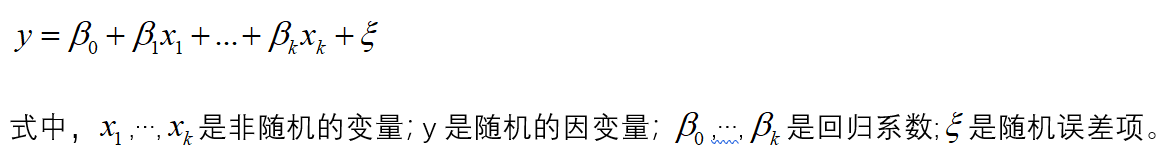如果对y和x进行了x次观测，得到n组观察值yi,x1i,…,xki(i=1,2,…,n),他们满足一下关系式：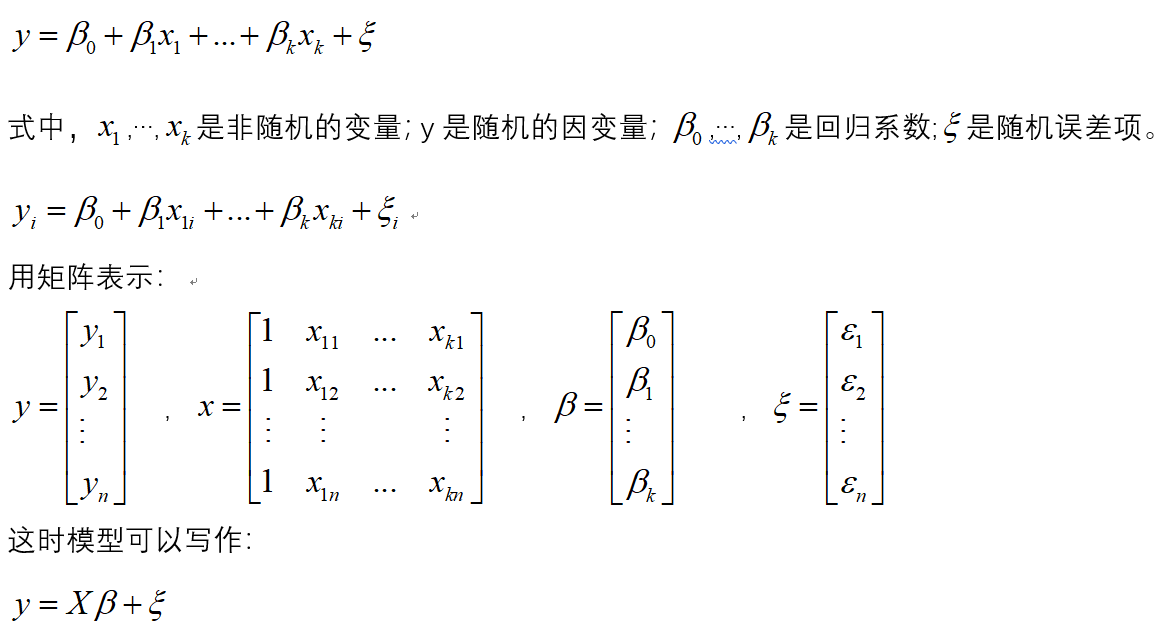1.2模型参数的检验
在正态假定下，如果X是列满秩的，则普通线性回归模型的参数最小二乘估计为：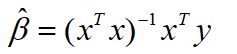于是y的估计值为：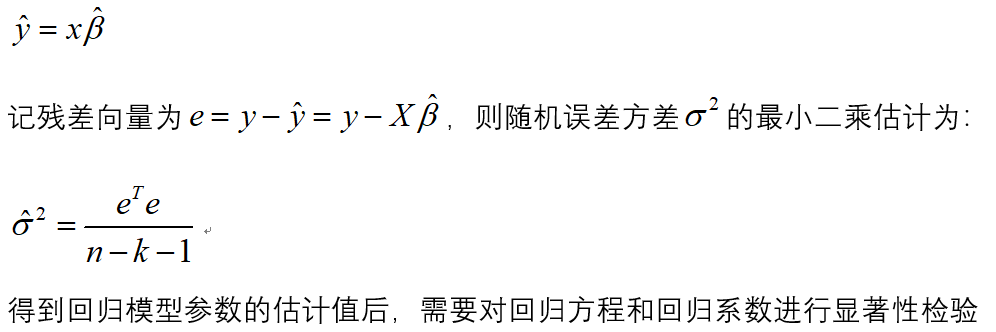（1）回归方程的显著性检验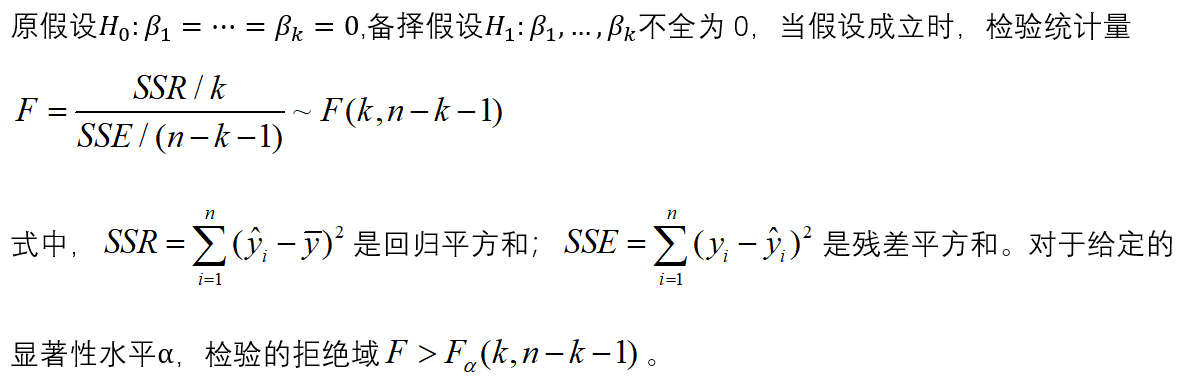（2）回归系数的显著性检验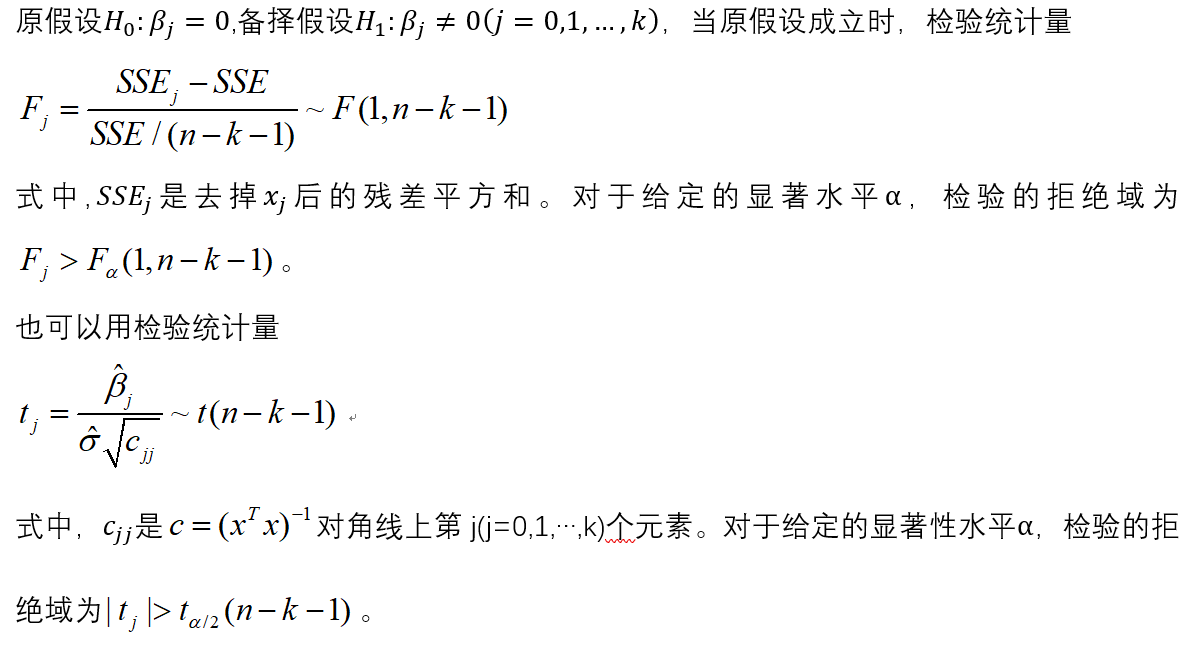2.建模步骤
（1）根据数据建立回归模型
（2）对模型进行显著性检验
（3)  对模型进行回归诊断
3.建模
library(car)
a
lm.salary=lm(锘縴~x1+x2+x3+x4,data=a)
summary(lm.salary)
#注：锘縴是y乱码之后的结果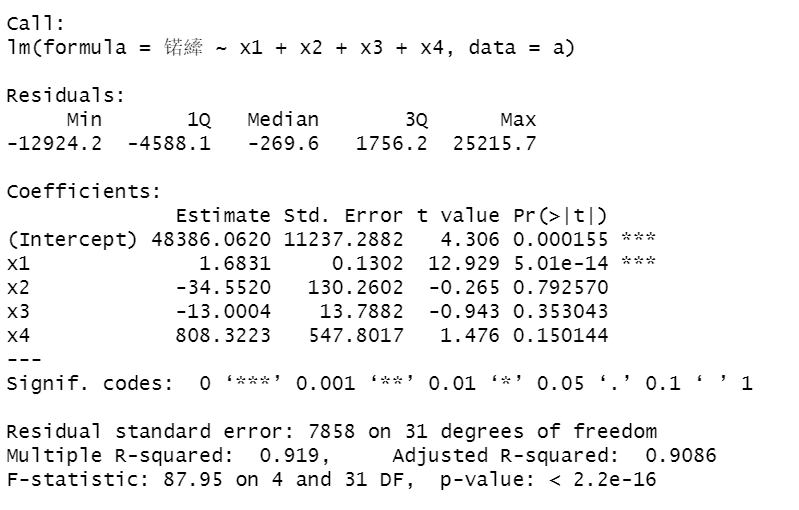发现x2,x3,x4系数不显著。
（2）对变量进行选择
lm.step=step(lm.salary,direction="both")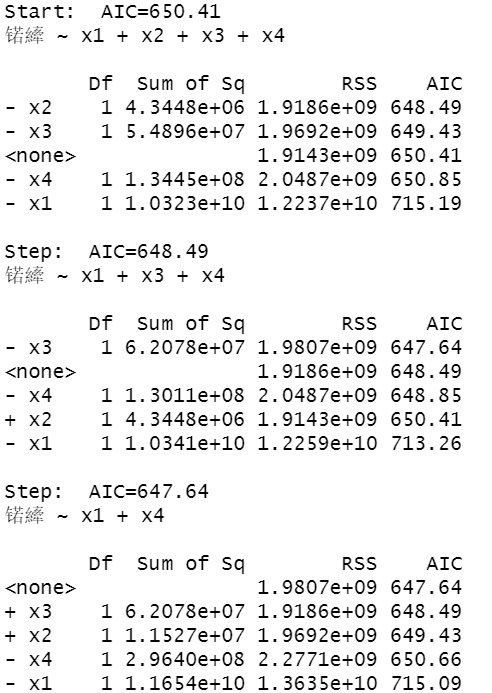如果去掉变量x2,AIC的值为648.49，如果去掉变量x3,AIC的值为650.85，如果去掉变量x1,AIC的值为715.19，所以在这里去掉x2.
进行下一轮的计算：
lm.salary=lm(锘縴~x1+x3+x4,data=a)
lm.step=step(lm.salary,direction="both")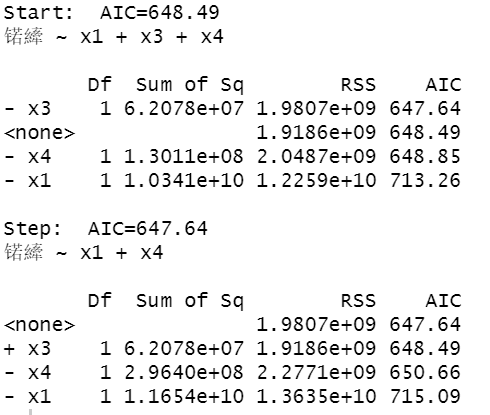发现去掉x3，AIC 的值为647.64，所以去掉x3.
单独对x1和x4，进行拟合。
lm.salary=lm(锘縴~x1+x4,data=a)
summary(lm.salary)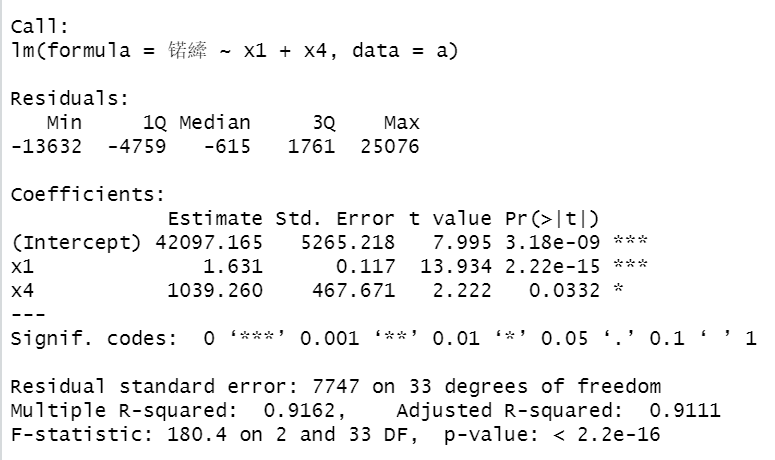可以看出F检验P值小于0.05显著，各个参数系数也是显著的。
（3）对上述回归模型进行回归残差诊断
算出模型的标准化残差
library(TSA)
y.rst=rstandard(lm.step)
y.rst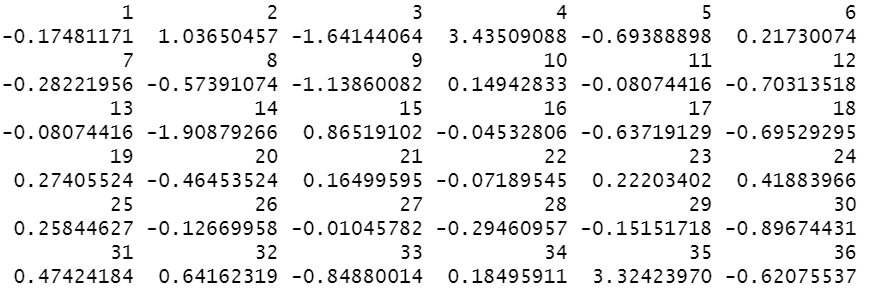画出其残差散点图：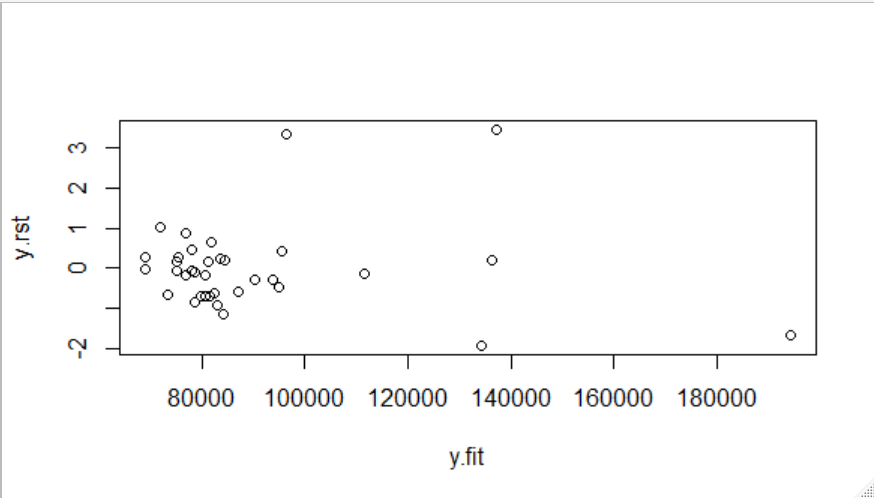很明显发现4和35号点异常，将这两个点去除。
lm.salary=lm(log(锘縴)~x1+x2+x3+x4,data=a[-c(4,35),])
lm.step=step(lm.salary,direction="both")
y.rst=rstandard(lm.step)
y.fit=predict(lm.step)
plot(y.rst~y.fit)

去除两点后的结果：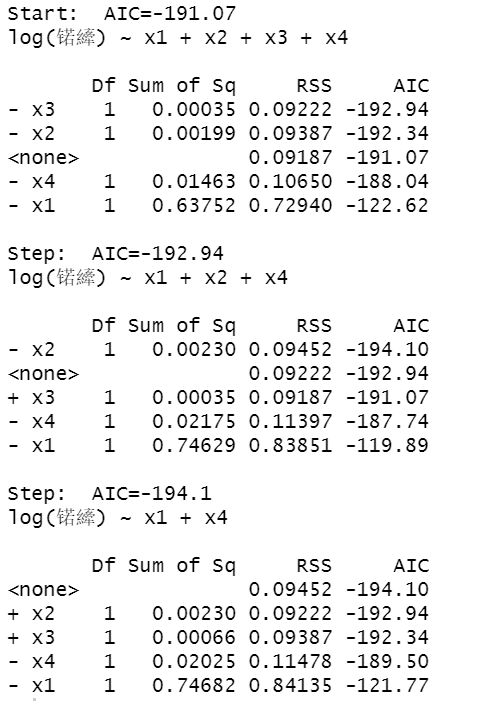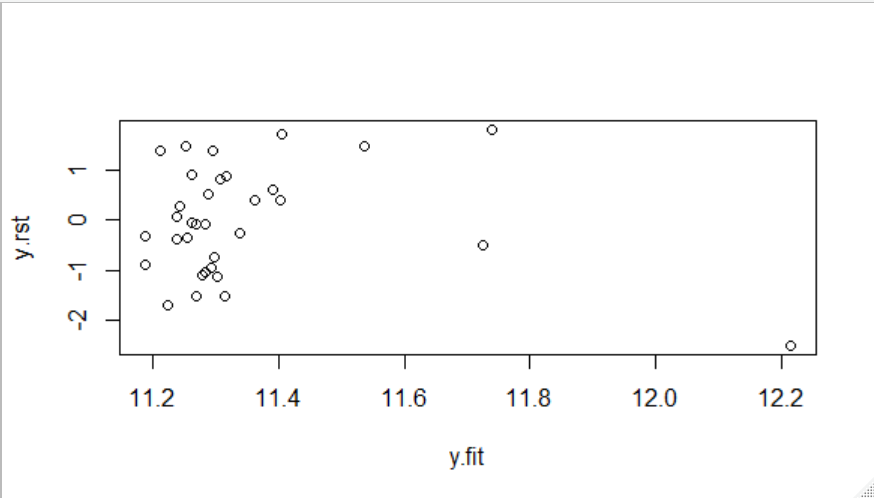绘制模型诊断图：
par(mfrow=c(2,2))
plot(lm.step)
influence.measures(lm.step)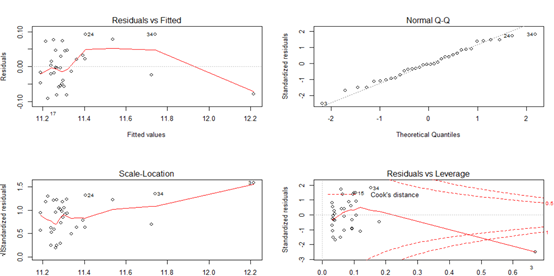残差拟合图基本上呈现随机分布模式，正态Q-Q图基本落在直线上，表明残差服从正态分布；大小-位置图和残差-杠杆图以小组的形式存在并且离中心不远。这说明3，4，35号观测值可能是异常点和强影响点。


展开全文• 本课程为基于Python的数据分析和统计建模高级课程，针对多元线性回归模型的各种参数和统计量，通过Python代码手工复现，在代码复现的过程中结合数学公式和原理进行深入解读。这些统计量包括：回归系数参数估计、决定...统计量 最小二乘法 偏度与峰度
• 终于找到一篇全面而又简洁的讲多元线性回归模型检验方法的文章 PDF下载地址 链接：https://pan.baidu.com/s/1UbyZcMC1VRTmlCEaX4Vybg 提取码：g481 具体内容 一、经济意义检验 经济意义检验主要检验模型参数估计量在...
终于找到一篇全面而又简洁的讲多元线性回归模型检验方法的文章
PDF下载地址
链接：https://pan.baidu.com/s/1UbyZcMC1VRTmlCEaX4Vybg
提取码：g481
具体内容
一、经济意义检验
经济意义检验主要检验模型参数估计量在经济意义。其表现为检验求得的参数估计值的符号与大小是否合理，是否与根据人们的经验和经济理论所拟定的期望值相符合。如果不符，则要查找原因和采取必要的修正措施，重新建立模型。
二、统计检验
1.拟合优度检验(${R^2}$检验)
拟合优度检验是检验回归方程对样本观测值的拟合程度，即检验所有解释变量与被解释变量之间的相关程度。
2.方程显著性检验(F检验)
方程显著性检验就是对模型中解释变量与被解释变
量之间的线性关系在总体上是否显著成立作出推断。即
检验被解释变量Y与所有解释变量戈l，石2，……，菇^之间
的线性关系是否显著，方程显著性检验所应用的方法是
数理统计学中假设检验。
3.变量显著性检验(t检验)
R2检验和F检验都是将所有的解释变量作为一个整体来检验它们与被解释变量Y的相关程度以及回归效果，但对于多元回归模型，方程的总体显著性并不意味每个解释变量对被解释变量Y的影响都是显著的。如果某个解释变量并不显著，则应该从方程中把它剔除，重新建立更为简单的方程。所以必须对每个解释变量进行显著性检验。
三、计量经济学检验
计量经济学检验是由计量经济学理论决定的，目的
在于检验模型的计量经济学性质。通常检验准则有随机
误差项的序列相关检验和异方差性检验，解释变量的多
重共线性检验等，其中最常用的是随机误差项的序列相
关检验。
在回归分析法中，假设随机误差项在不同的样本点
之间是不相关的，即si与8i(i≠_『)相互独立。但在实际
问题中，经常出现与此相违背的情况，占i与si(i≠．『)之
间存在相关性，称为序列相关。若存在序列相关，则此时
的回归模型无效，必须重新建立回归模型。
在序列相关中，最常见的是一阶自相关即占i与sf+l
相关，而对一阶自相关最常用的检验方法是DW检验法
模型预测检验
预测检验主要检验模型参数估计量的稳定性以及相对样本容量变化时的灵敏度，确定所建立的模型是否可以用于样本观测值以外的范围，即模型的所谓超样本特性。具体检验方法为：
①利用扩大了的样本重新估计模型参数，将新的估计值与原来的估计值进行比较，并检验二者之间差距的显著性。
②将所建立的模型用于样本以外某一时期的实际预测，并将该预测值与实际观测值进行比较，并检验二者之间差距的显著性。


展开全文F检验 t检验
• 文章目录1. 前文回顾2. 最小二乘法估计（Ordinary Least Squared, OLS）3. 极大似然估计...在上一篇文章中，我们建立了多元回归正 yi=β0+∑j=1pxijβj+ϵi, i=1,...,N y_i= \beta_0 + \sum_{j=1}^...
文章目录1. 前文回顾2. 最小二乘法估计（Ordinary Least Squared Estimate, OLS）3. 极大似然估计（Maximum Likelihood Estimate, ML）4. 结论5. 新问题的提出参考文献写在最后
【更新日志】
3/3/2020 对部分公式中出现的错误进行了修正
4/5/2020 修改了文章标题

1. 前文回顾
在上一篇文章中，我们建立了多元线性回归模型，以及模型的相关假设，并给出了对应的样本模型（详情请见：【统计学习系列】多元线性回归模型（一）——模型的建立与基本假设）（别问我为什么点开404，因为这一章的内容太多我还在补充中，暂未开放）：
$y_i= \beta_0 + \sum_{j=1}^{p} x_{ij} \beta_j + \epsilon_i , \ i=1,...,N$其中：
$\bm{\epsilon} = (\epsilon_i)_{N \times 1} \thicksim N(0,\bm{I_n}\sigma^2)$
因此，需要估计的参数有 p + 2 个：β0, β1, …,  βp, σ。我们如何利用样本来估计模型参数呢？我们的一般思路是：1）制定评价标准，2）在给定标准下寻找最优参数。这篇文章主要介绍最小二乘估计法和极大似然估计法这两种方法。话不多说，让我们开始探寻参数估计的秘密吧！
注：本篇涉及大量数学定理推导。尽管作者力求内容通俗易懂，但同时也希望保证证明的严谨性。因此在参数估计的推导中，我会把证明思路与过程尽可能清晰、完整地展示出来，这可能需要读者具有一定程度的凸优化问题求解和线性代数的基础。实操应用类读者可直接跳过2、3两章，而直接阅读第4章结论。

2. 最小二乘法估计（Ordinary Least Squared Estimate, OLS）
如果将 N 组样本对 (yi, xi) 看做是一个 p+1 维实空间中的N个点，那么我们现在要做的就是在空间中找到一个 p 维超平面，来尽可能“好”的拟合空间中的这N个样本的点。什么样的指标可以衡量这种拟合的好坏呢？样本点到拟合平面的距离则是一个衡量拟合好坏的测量工具：当点到平面距离和越小，说明估计量与真实值之间的“距离”越小（离得越近），模型对样本数据的拟合情况越好；而点到平面距离和越大，说明估计量与真实值之间的“距离”越大（离得越远），模型对样本数据的拟合情况越差。而对于距离的度量，我们可以使用两点差值的平方这一指标：
$distance(y_i, \hat{y}_i) = (y_i - \hat{y}_i)^2$
注1：使用平方而非绝对值是为了后边方便求导。
注2：这里的表述并不严谨。作为距离的测度，我们应该使用范数（例如2-范数）。其本质是因为定义范数作为距离的欧式空间是一个赋范线性空间。
最小二乘估计法的核心思想是：找到一组参数 β ，使得“样本点到平面的距离和最小”，或者说最小化残差平方和。用数学语言可以表示为：
$\min_{\beta_0, \beta_1,...,\beta_p} RSS(\beta_0, \beta_1,...,\beta_p) \\ \hskip{1.5em} = \sum_{i=1}^{N}(y_i - f(\bm{x_i} ))^2 \\ \hskip{5em} = \sum_{i=1}^{N}(y_i - \beta_0 - \sum_{j=1}^{p} x_{ij} \beta_j)^2$
注1：利用这一准则成立的合理前提是：(xi, yi)是从总体一种独立抽取的随机样本。若样本不满足随机性，yi与xi也应该条件独立。
注2：在利用这一准则进行参数估计时，其过程本身并不蕴含任何假设条件（即该过程并不蕴含模型的有效性假设）
$RSS( \bm{\beta} ) = (\bm{y} - \bm{X} \bm{\beta} )^T (\bm{y} - \bm{X} \bm{\beta} )$
其中：
$\bm{\beta} = \begin{bmatrix} \beta_0 \\ \beta_1 \\ \vdots\\ \beta_p \end{bmatrix}_{(p+1) \times1}, \space \space \space \space \space \bm{y} = \begin{bmatrix} y_0 \\ y_1 \\ \vdots\\ y_N \end{bmatrix}_{N \times1}, \space \space \space \space \space \bm{X} = \begin{bmatrix} \space 1 \space\space x_{11} \space\space \dots \space\space x_{1p} \\ \space 1 \space\space x_{21} \space\space \dots \space\space x_{2p} \\ \vdots \space \space\space \space \space \vdots \space \space \space \space \space ⋱ \space \space \space \space \vdots\\ \space 1 \space\space x_{N1} \space\space \dots \space\space x_{Np} \end{bmatrix}_{N \times (p+1)}$
由于问题（I）可以视为无约束优化问题，分别对目标函数求其一阶和二阶导数，则有：
$\frac{ \partial RSS( \bm{\beta} ) } { \partial \bm{\beta} } = -2 \bm{X}^T ( \bm{y} - \bm{X} \bm{\beta})$$\frac{ \partial^2 RSS( \bm{\beta} ) } { \partial \bm{\beta}^2 } = 2 \bm{X}^T \bm{X} \succcurlyeq 0$
根据上述结论，该最优化问题的解满足：$\bm{X}^T ( \bm{y} - \bm{X} \bm{ \hat\beta}) = 0$
移项得：$\bm{X}^T \bm{X} \bm{\hat\beta} = \bm{X}^T \bm{y}$
当自变量不存在多重共线性时， XTX 矩阵非奇异，因此其逆矩阵存在，因此解得优化问题的解：
$\bm{\hat\beta} = ( \bm{X}^T \bm{X} )^{-1} \bm{X}^T \bm{y}$
注1：矩阵 XTX 非奇异， 等价于 样本资料阵 X 列满秩，等价于模型自变量不存在多重共线性。这部分证明请参考文献《高等代数（第二版）上册 》 ；
注2：关于矩阵函数的代数运算，例如乘法运算、逆运算、求导运算等更多细节，请参考文献《高等代数（第二版）上册 》 ；
注3：关于凸规划问题的相关基础知识，请参考文献《数值最优化》 。
将估计参数 β 带入模型中，可以得到学习样本的拟合值：
$\bm{\hat y} = \bm{X} \bm{\hat\beta} = \bm{X} ( \bm{X}^T \bm{X} )^{-1} \bm{X}^T \bm{y} \xlongequal{def} \bm{H} \bm{y}$其中，矩阵 H ：$\bm{H} = \bm{X} ( \bm{X}^T \bm{X} )^{-1} \bm{X}^T$被称为“帽子矩阵”（仿佛给 y 带了一个帽子）。
参数 σ 表示误差项的波动率。我们可以把残差序列作为误差项的估计量，因此可以使用残差序列的样本标准差对 σ 进行估计：
$\bm{\hat \sigma} = \sqrt{ \frac {( \bm{y} - \bm{\hat{y}} )^T ( \bm{y} - \bm{\hat{y}} ) } {N-p-1} } = \sqrt{ \frac { \sum _{i=1}^{N} (y_i - \hat{y}_i)^2} {N-p-1} }$
注：这里分母使用 N-p-1 是为了确保该方差估计量的无偏性，其具体论证我将在下一篇文章中进行说明。

3. 极大似然估计（Maximum Likelihood Estimate, ML）
在上一章使用最小二乘法对模型参数进行估计时，我们采用了用“残差平方和”作为评判模型拟合的好坏，其实质是希望找到一个拟合超平面（即为一个线性模型），使得所有样本到超平面的距离和最短（也就是最接近）。因此，这种评估方法是站在几何学的角度上进行的。除此之外，我们还有没有其他衡量拟合好坏的角度呢？
首先我们知道，样本具有“二元性” ：当在抽样之前，样本可以视为一个 随机变量（或随机向量）；而抽样之后，样本的取值被固定，因此又可以视为一个常数（或常向量）。因此，在抽样之前，N 个来自于总体的独立随机样本应该有联合分布，这是随机变量的特征决定的。若多元线性回归模型的正态性假设成立，那么N 个样本的因变量 y 的条件分布应满足：
$\bm{y} \thicksim N(\bm{X} \bm{\beta},\bm{I_n}\sigma^2)$
其联合概率密度函数存在，有：
$f ( y_1, ..., y_N; \bm{x_1} , ..., \bm{x_N}, \bm{\beta }, \sigma ) \\ \hspace{4.5em} = \prod_{i=1}^{N} \frac {1} {\sqrt{2\pi}\sigma} \exp\{ - \frac{1}{2\sigma^2} (y_i - \beta_0 - \beta_1 x_{i1}- \dots - \beta_p x_{ip})^2 \} \\ \hspace{4.5em} = \frac {1} { ( \sqrt{2\pi}\sigma ) ^ N} \times \exp\{ - \sum_{i=1}^N \frac{1}{2\sigma^2}(y_i - \beta_0 - \beta_1 x_{i1}- \dots - \beta_p x_{ip} ) ^2 \} \\ \hspace{4.5em} =( \sqrt{2\pi}\sigma ) ^ {-N} \times \exp \{ - \frac{1}{2\sigma^2} (\bm{y} - \bm{X} \bm{\beta} )^ T(\bm{y} - \bm{X} \bm{\beta} ) \}$其中： φ(x) 为一元标准正态分布的分布函数。
注：关于随机变量与分布更多的资料请参考文献《测度论与概率论基础》
在抽样之后，样本被固定，联合概率密度函数变成了关于总体参数 β 的函数。我们重新定义这个函数，称这一函数为“似然函数”（Likelihood Function），并记为 L (β)，其表达式为：
$L( \bm{\beta } , \sigma; \space (y_1, \bm{x_1}), ..., (y_N, \bm{x_N}) ) =( \sqrt{2\pi}\sigma ) ^ {-N} \times \exp \{ - \frac{1}{2\sigma^2} (\bm{y} - \bm{X} \bm{\beta} )^ T(\bm{y} - \bm{X} \bm{\beta} ) \}$
注：似然函数与联合概率密度函数在表达形式上一致，但是似然函数是关于总体参数 β 的函数，而概率密度函数是关于随机变量序列（即样本序列）( yi, xi ), i = 1, …, N 的函数。
为什么称他为似然函数呢？我们知道，概率密度函数展现了的随机变量发生的可能性的大小。因此似然函数的意义是：若我们已经抽出来了一组样本，那么这组样本来自参数为 β 的总体的可能性是多少？
注：这个说法不够严谨，但这是我能想到的最容易理解的表达了。。。
那么我们如何利用似然函数来估计参数呢？我们期望：“在一次试验中，若某一事件发生了，那么这一事件最有可能来自于发生可能性最大的哪一种情形”，或者说“发生概率最高的事件在一次实验中最有可能发生”。
这听起来完全就是废话啊！我们不妨举一个简单的例子：
例：一个箱子里有十个球，这十个球有可能是下面三种情况：
情况 1：十个球中，一个红球，九个白球；
情况 2：十个球中，五个红球，五个白球；
情况 3：十个球中，九个红球，一个白球；
已知有放回地随机抽，每次抽一个，结果三次都抽中了红球。请问，箱子里最有可能是这三种情况中的哪一种？
分别计算这三种情况所发生“有放回三次都抽中红球”这一事件的概率，可以知道情况3发生的可能性最大。因此我们可以认为箱子里装有九个红球和一个白球。
注：对一思想进行严格说明需要涉及贝叶斯决策，有兴趣的读者可自行查阅更多资料
通过这个例子的说明可知：我们需要找到一组参数，使得在这组参数下，样本的联合概率密度达到最大。因此，这个问题就变成了：在所有有可能的参数取值中（专业说法：在参数空间中），所抽中的样本来自哪一组参数的可能性最高？
至此，我们将问题抽象成了一个无约束优化问题，这与最小二乘估计的手段十分相似。但是由于似然函数是连乘运算，这在求导的过程中不够方便，因此我们对似然函数其进行对数化处理，得到“对数似然函数”（Logarithm Likelihood Function ），并记为 l (β)。因此，这一优化问题转化为了：
$\min_ \bm{\beta} l( \bm{\beta }, \sigma;\space (y_1, \bm{x_1}), ( y_2, \bm{x_2}), ... , (y_N, \bm{x_N}) ) = -N \ln { \sqrt{2\pi}\sigma } - \frac{1}{2\sigma^2} ( \bm{y} - \bm{X} \bm{\beta} )^ T(\bm{y} - \bm{X} \bm{\beta} )$
注：不难证明：对数似然函数与似然函数取最小值时，β 相等。
与第2部分过程相仿，其一阶导函数有：
$\frac{ \partial l( \bm{\beta},\sigma ) } { \partial \bm{\beta} } = -2 \bm{X}^T ( \bm{y} - \bm{X} \bm{\beta}) = 0$$\frac{ \partial l( \bm{\beta},\sigma) } { \partial \sigma } = -\frac{N}{\sigma} + \frac{1}{\sigma^3} ( \bm{y} - \bm{X} \bm{\beta} )^ T(\bm{y} - \bm{X} \bm{\beta} ) = 0$
解得：
$\bm{\hat\beta} = ( \bm{X}^T \bm{X} )^{-1} \bm{X}^T \bm{y}$$\hat{\sigma} = \sqrt { \frac{1}{N} ( \bm{y} - \bm{X} \bm{\beta} )^ T(\bm{y} - \bm{X} \bm{\beta} ) }$

4. 结论
基于第2部分和第3部分的讨论，我们知道：基于最小二乘法和极大似然估计法两种方法得到的参数估计结果是一致的（有没有一种殊途同归的艺术感！），估计值为：
$\bm{\hat\beta} = ( \bm{X}^T \bm{X} )^{-1} \bm{X}^T \bm{y}$
其中：
$\bm{\beta} = \begin{bmatrix} \beta_0 \\ \beta_1 \\ \vdots\\ \beta_p \end{bmatrix}_{(p+1) \times1}, \space \space \space \space \space \bm{y} = \begin{bmatrix} y_0 \\ y_1 \\ \vdots\\ y_N \end{bmatrix}_{N \times1}, \space \space \space \space \space \bm{X} = \begin{bmatrix} \space 1 \space\space x_{11} \space\space \dots \space\space x_{1p} \\ \space 1 \space\space x_{21} \space\space \dots \space\space x_{2p} \\ \vdots \space \space\space \space \space \vdots \space \space \space \space \space ⋱ \space \space \space \space \vdots\\ \space 1 \space\space x_{N1} \space\space \dots \space\space x_{Np} \end{bmatrix}_{N \times (p+1)}$
不过这两种方法对误差项方差的估计不同。最小二乘法对误差项标准差的估计量为：
$\bm{\hat \sigma} _{OLS} = \sqrt{ \frac {1 } {N-p-1} ( \bm{y} - \bm{\hat{y}} )^T ( \bm{y} - \bm{\hat{y}} ) }$
而极大似然估计对误差项标准差的估计量为：$\bm{\hat \sigma} _{ML} = \sqrt{ \frac { 1 } {N} ( \bm{y} - \bm{\hat{y}} )^T ( \bm{y} - \bm{\hat{y}} ) }$其中：$\bm{\hat y} = \bm{X} \bm{\hat\beta} = \bm{X} ( \bm{X}^T \bm{X} )^{-1} \bm{X}^T \bm{y}$
注：在机器学习领域中，我们首先设置目标函数（或称代价方程），通过寻找最优的参数使得目标函数达到最小值，从而实现参数估计的目的。因此从这个意义上讲，多元线性回归模型可以算机器学习模型中的一种。

5. 新问题的提出
在估计出了参数之后，小伙伴们 （无中生有） 一定会顺理成章地提出如下疑问：
（1）这些估计出来的参数好不好？准不准确呢？准确率有多高呢？
（2）估计出来的模型好不好？有没有效呢？
（3）估计出来的模型是否满足模型的基本假设条件呢？不满足可咋办呢？
（4）如何利用估计出来的模型进行预测呢？预测的准确性有多高呢？
（5）你还得拖多长时间才往后更新啊？
面对小伙伴们的灵魂拷问，让我们下回分解。

参考文献
《高等代数（第二版）上册 》丘维声 著
《数值最优化》李董辉等 著
《测度论与概率论基础》程士宏 著

写在最后
欢迎感兴趣的小伙伴来跟作者一起挑刺儿~ 包括但不限于语言上的、排版上的和内容上的不足和疏漏~ 一起进步呀！
有任何问题，欢迎在本文下方留言，或者将问题发送至勘误邮箱： mikeysun_bugfix@163.com
谢谢大家！XD


展开全文机器学习 统计学习
• 什么是回归分析？ 相关性 ≠ 因果性 ...[外链图片转存失败,源站可能有防盗链机制,建议将图片保存下来直接上传(img-NKvCz36V-1600444325643)(figures/多元线性回归模型/image-20200824222649255.
什么是回归分析？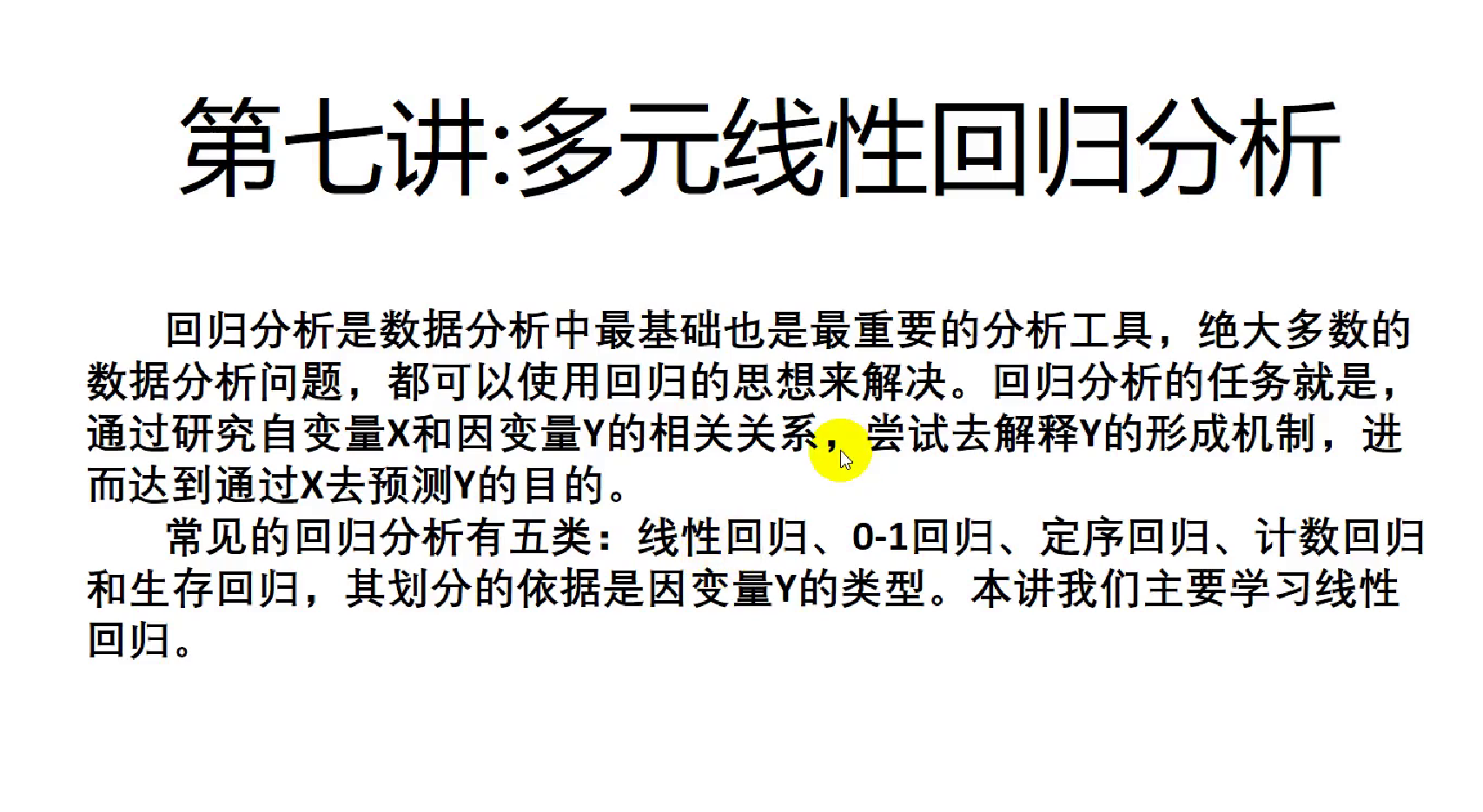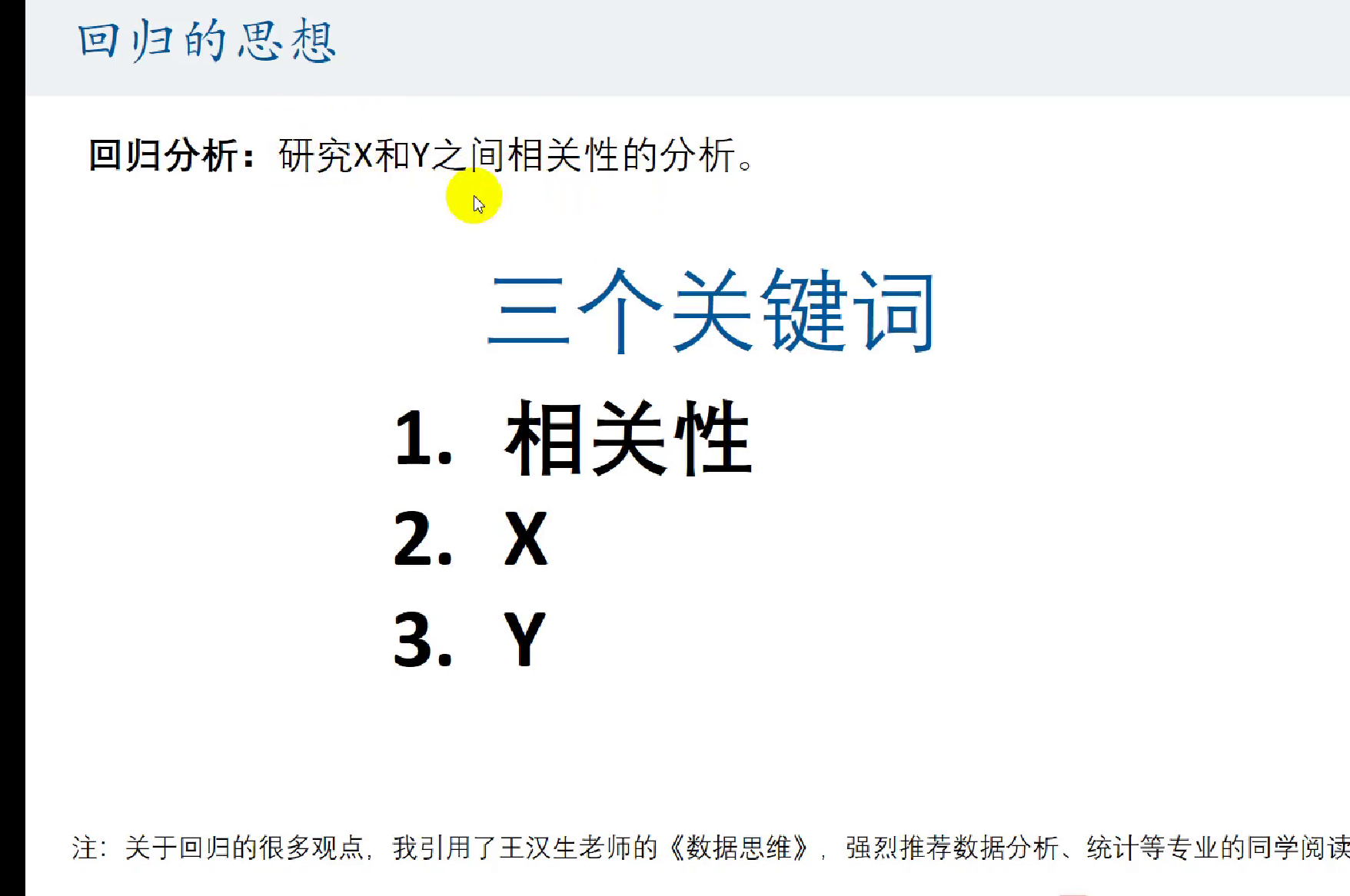相关性 ≠ 因果性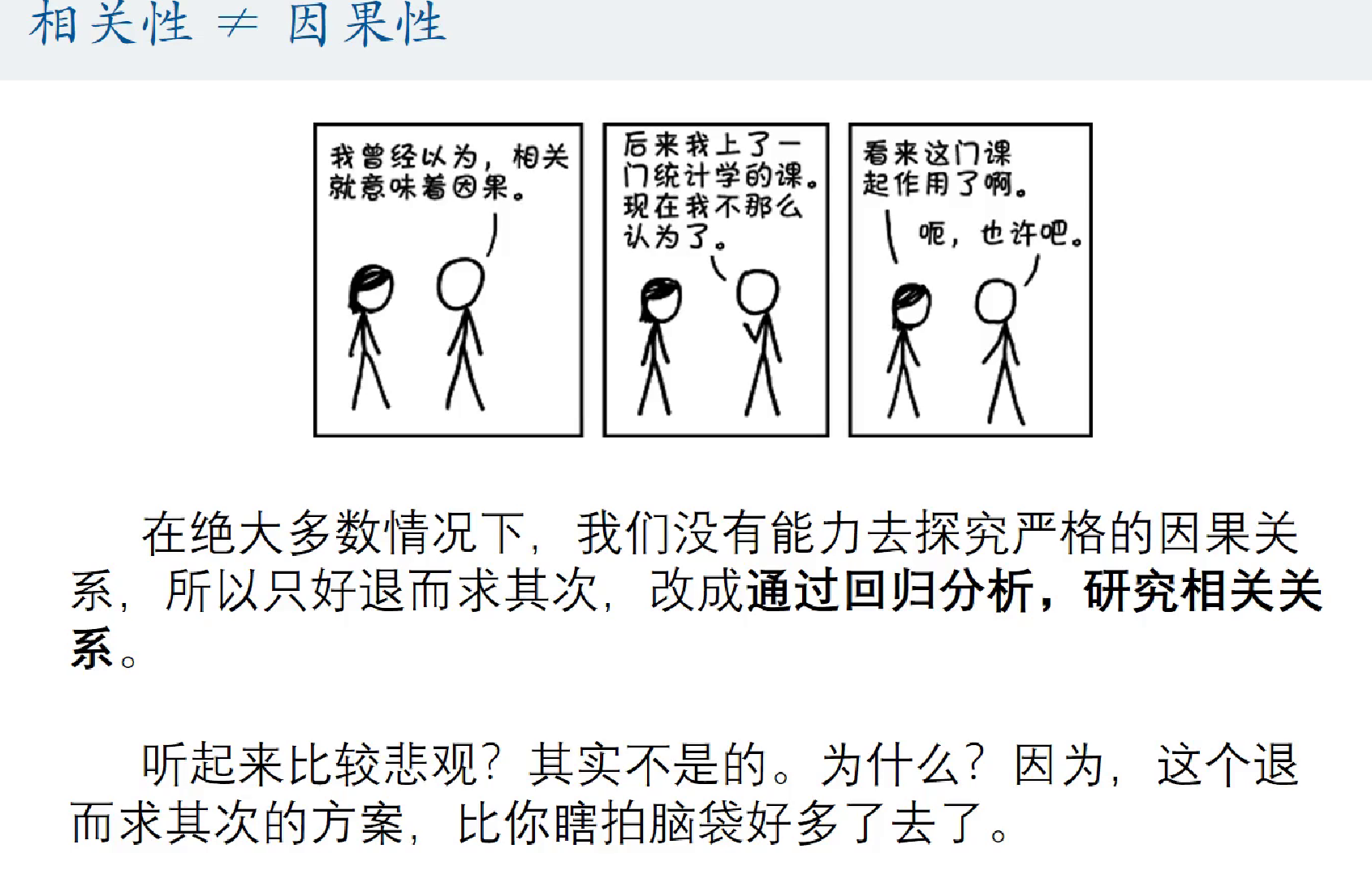自变量Y：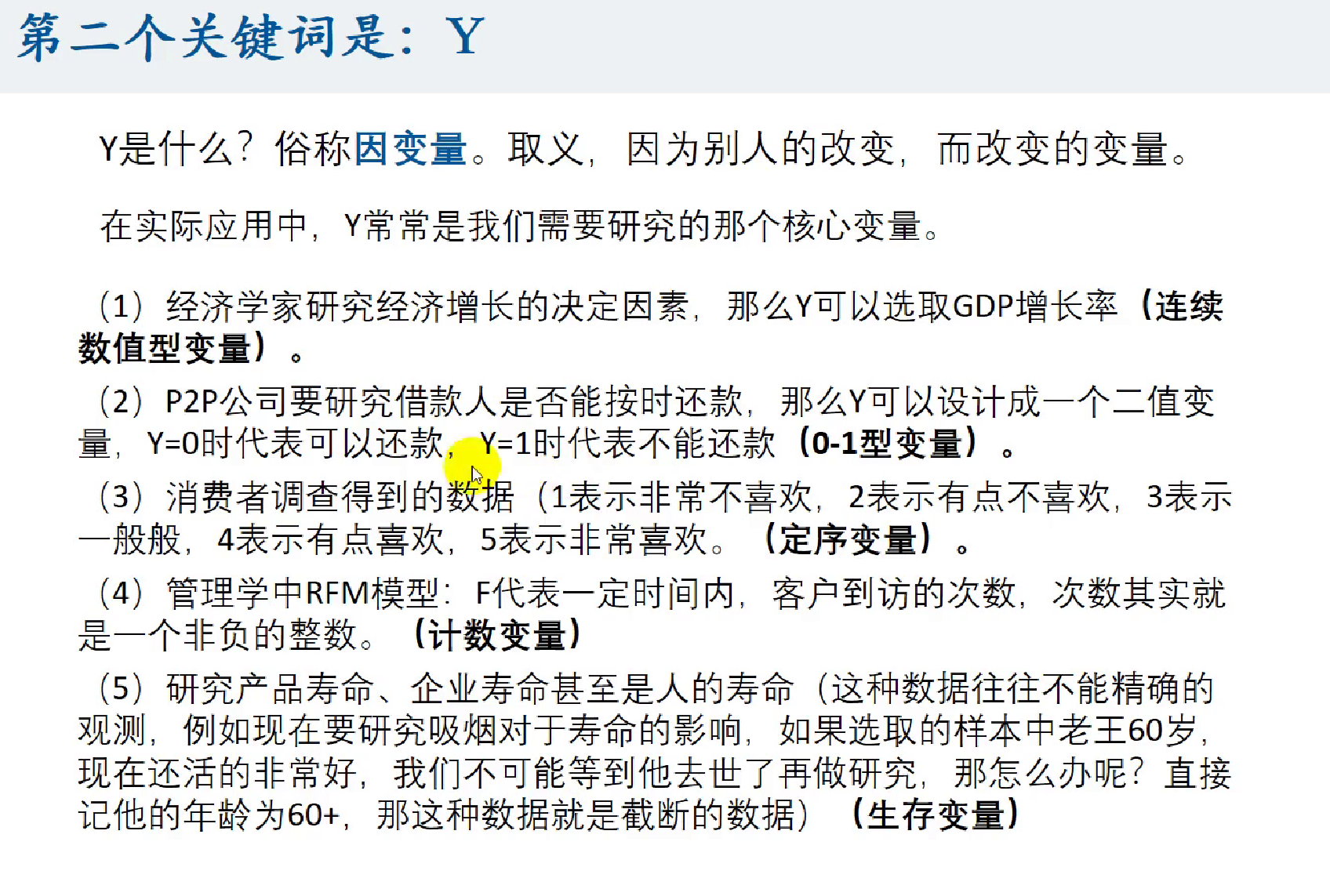自变量X：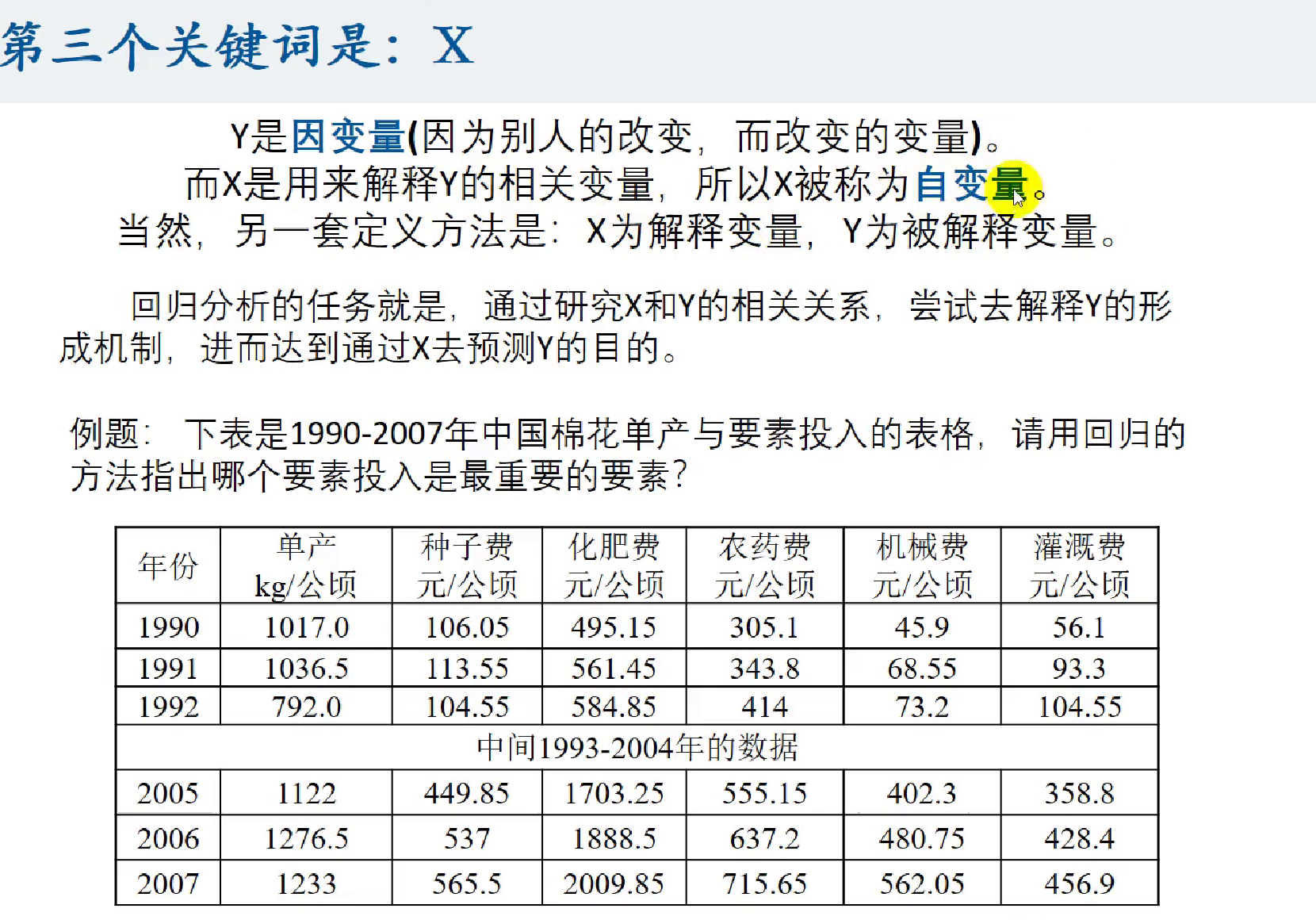回归分析的用处：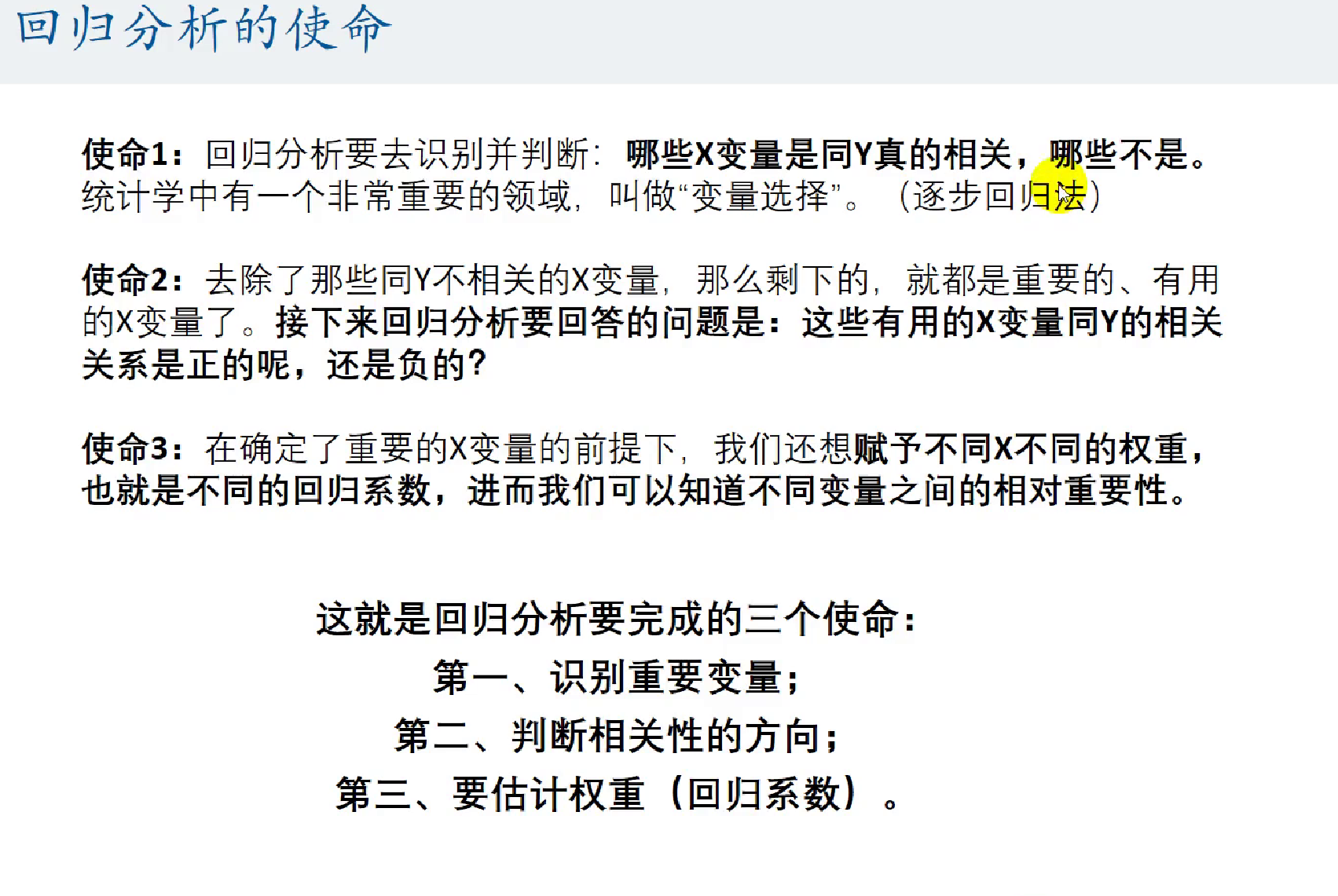这里要注意，因为涉及到不同自变量的权重，所以一般要去量纲，不然没意义。

回归分析的分类：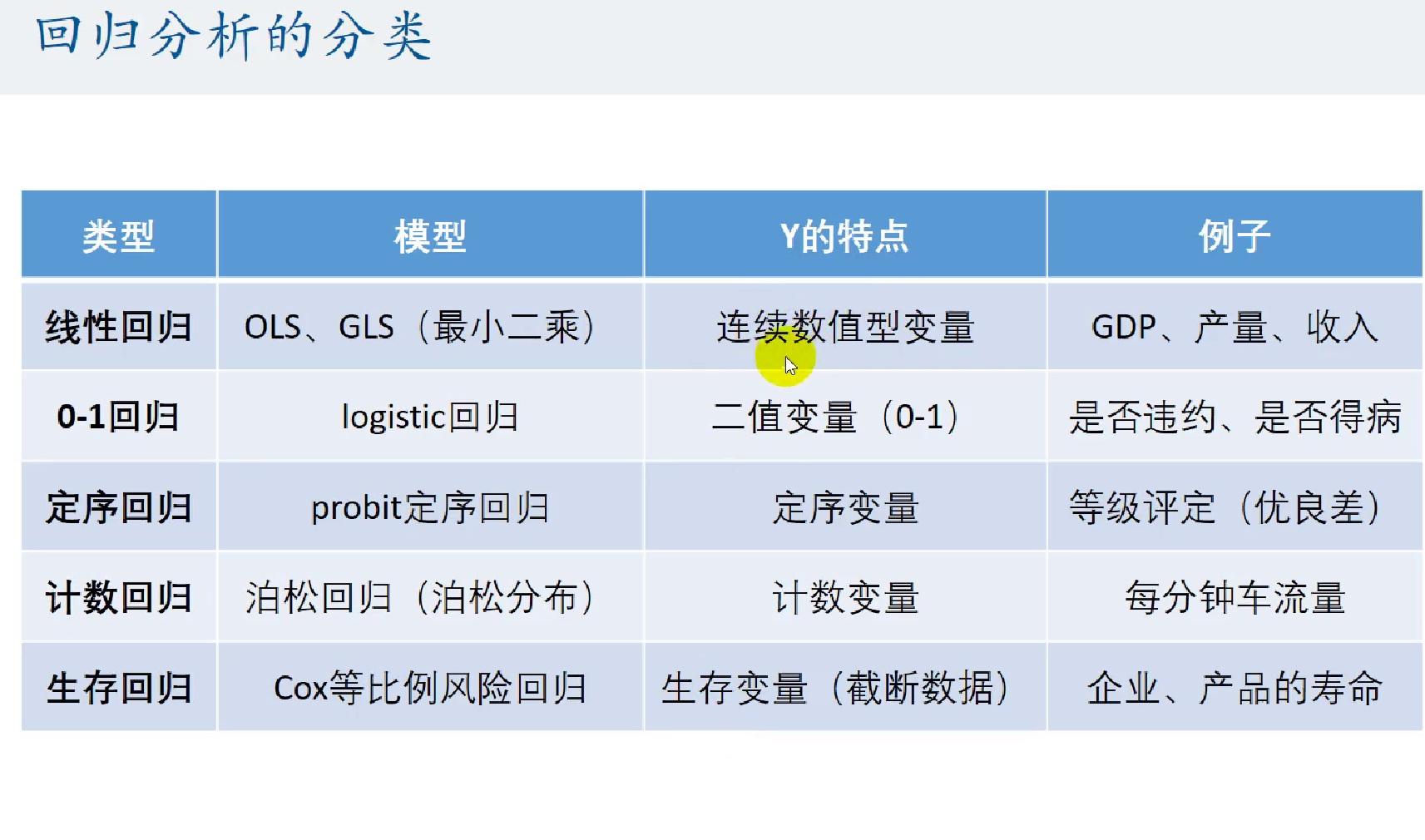多元线性回归：
不同数据的处理：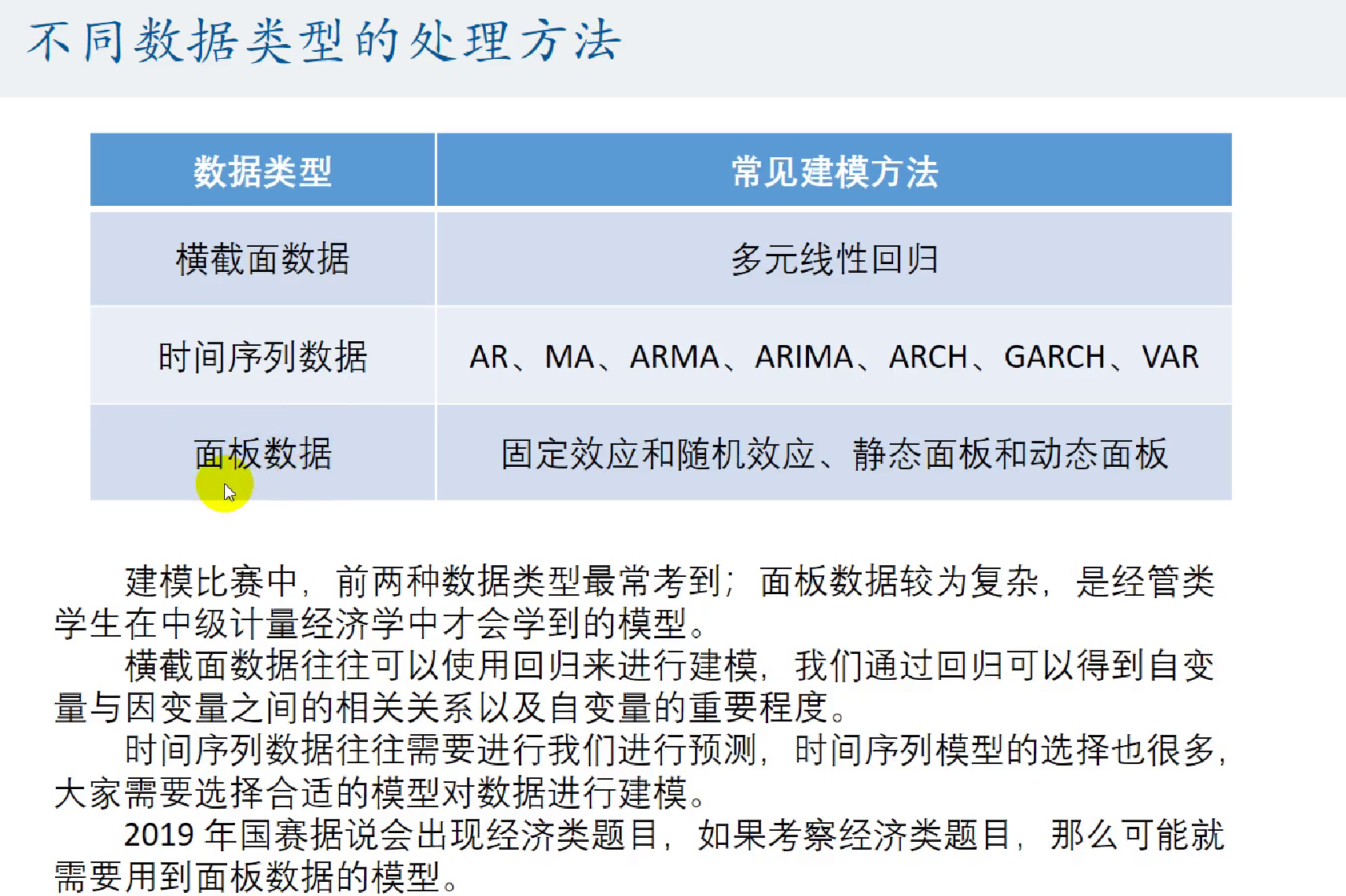横截面数据：多元线性回归
时间序列数据：最常用的是ARMA

横截面数据：
[外链图片转存失败,源站可能有防盗链机制,建议将图片保存下来直接上传(img-NKvCz36V-1600444325643)(figures/多元线性回归模型/image-20200824222649255.png)]
时间序列数据：面板数据：数据的收集：
【简道云汇总】110+数据网站
虫部落数据搜索
【汇总】数据来源/大数据平台
大数据工具导航工具(http://hao.199it.com/)
数据平台

上面的数据多半都是宏观数据，微观数据市面上很少
大家可以在人大经济论坛搜索
一元线性回归：
一元线性回归和一元线性函数拟合区别：

定义不一样，本质是一样的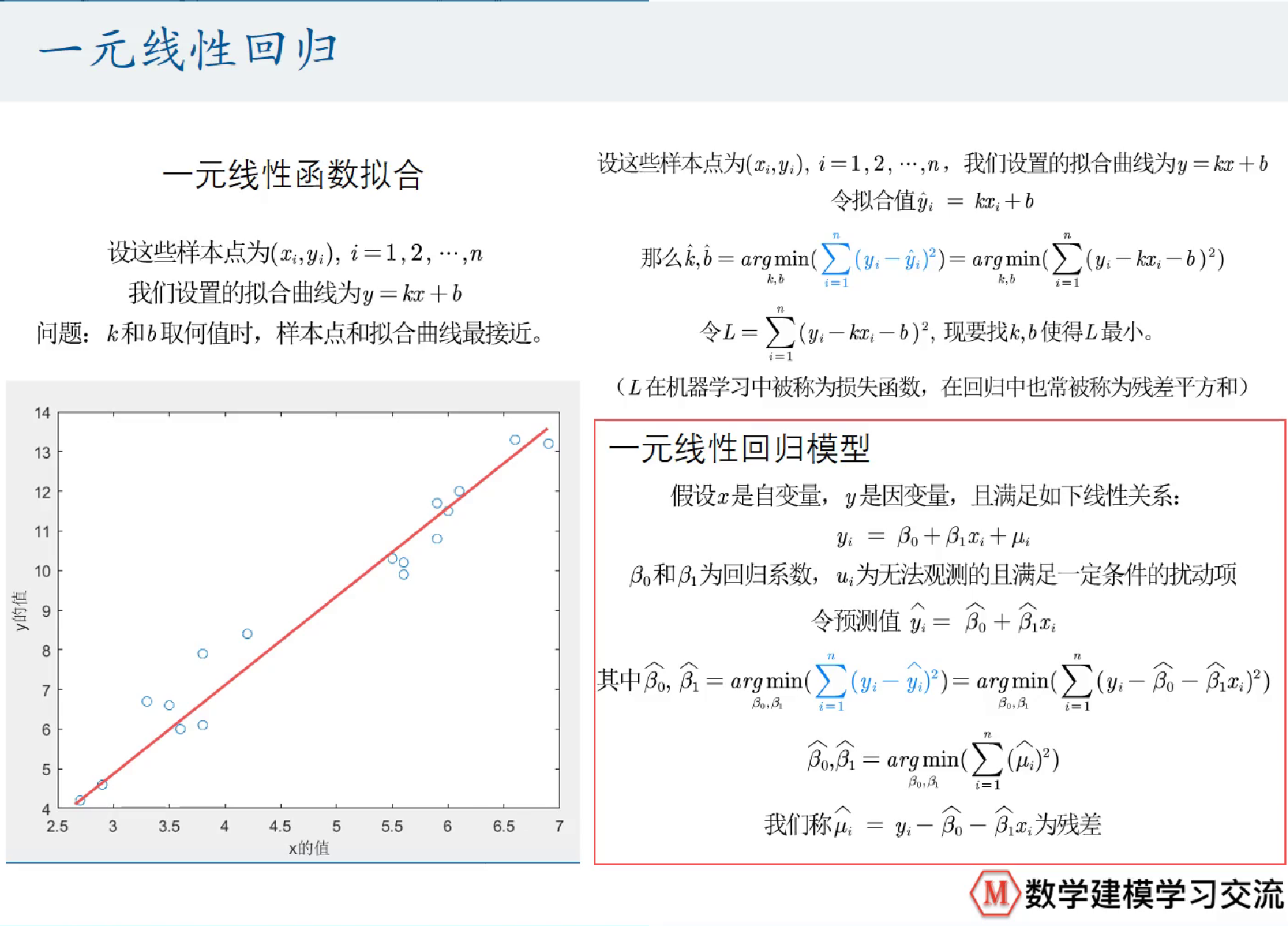对线性的理解：

线性是对参数说的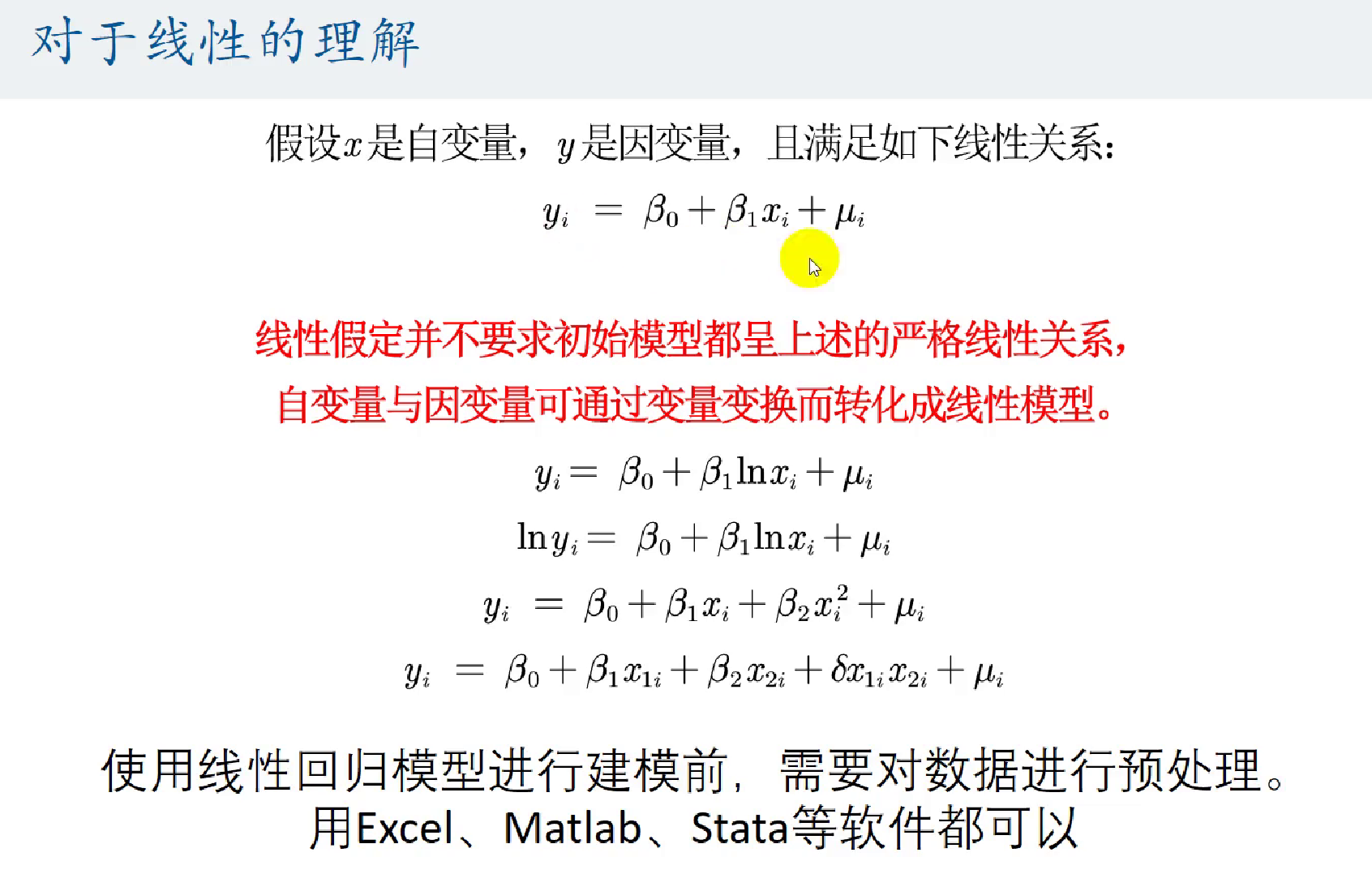回归系数的解释：

求系数很简单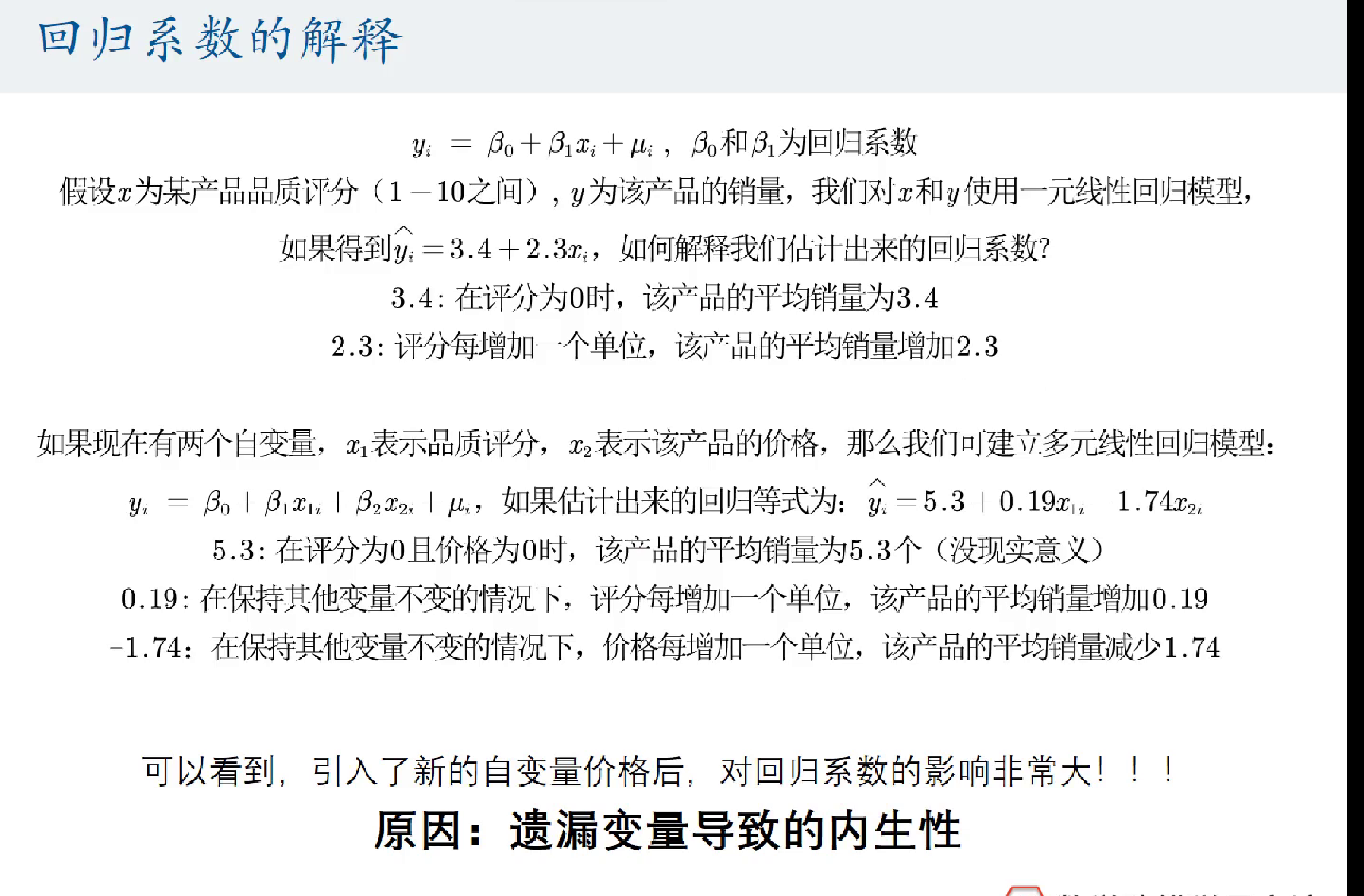这里的$y_i$的$i$是对每个个体而言，也可以去掉，转换成对总体而言

内生性：

无偏性和一致性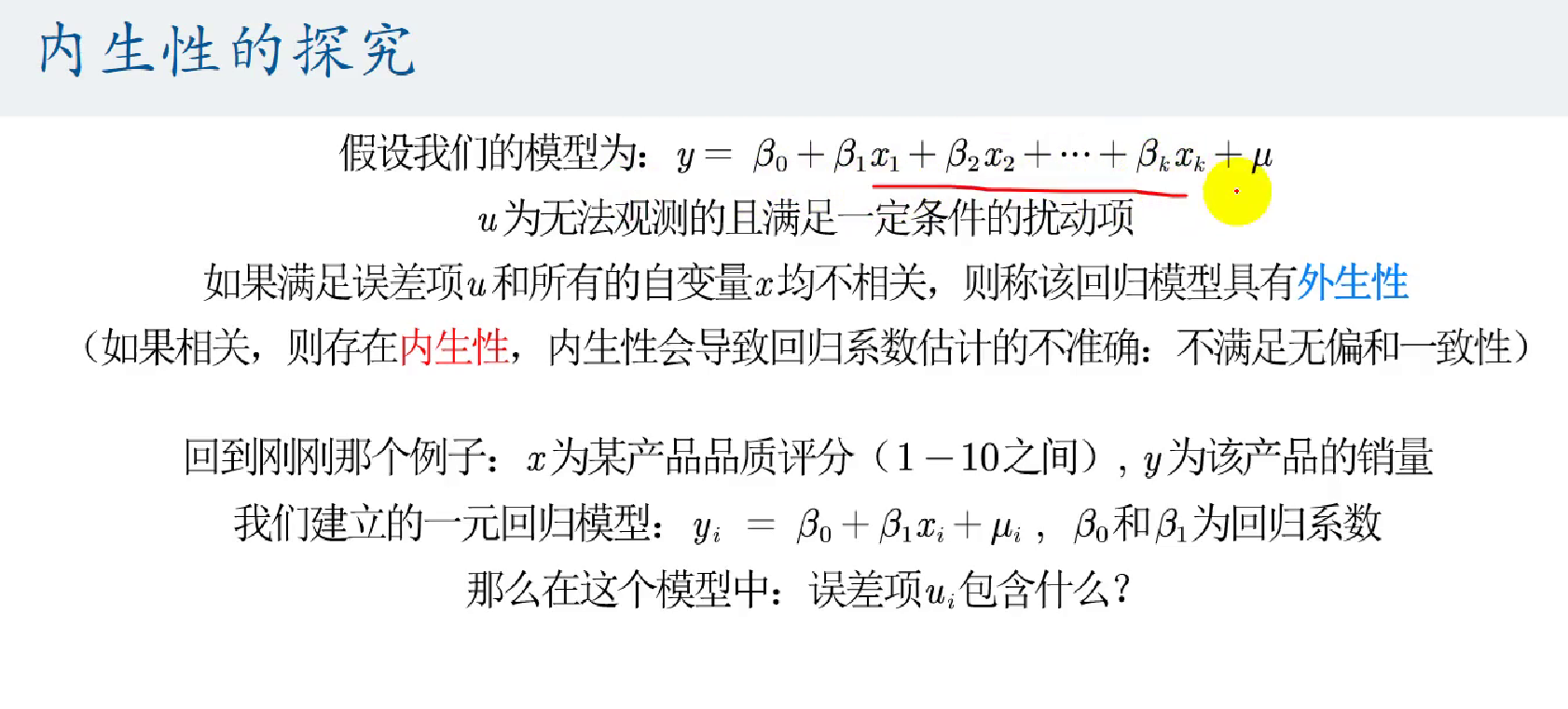$u_i$是什么？
[外链图片转存失败,源站可能有防盗链机制,建议将图片保存下来直接上传(img-LXnSvChO-1600444325652)(figures/多元线性回归模型/image-20200824235144988.png)]
蒙特卡洛验证是否有内生性：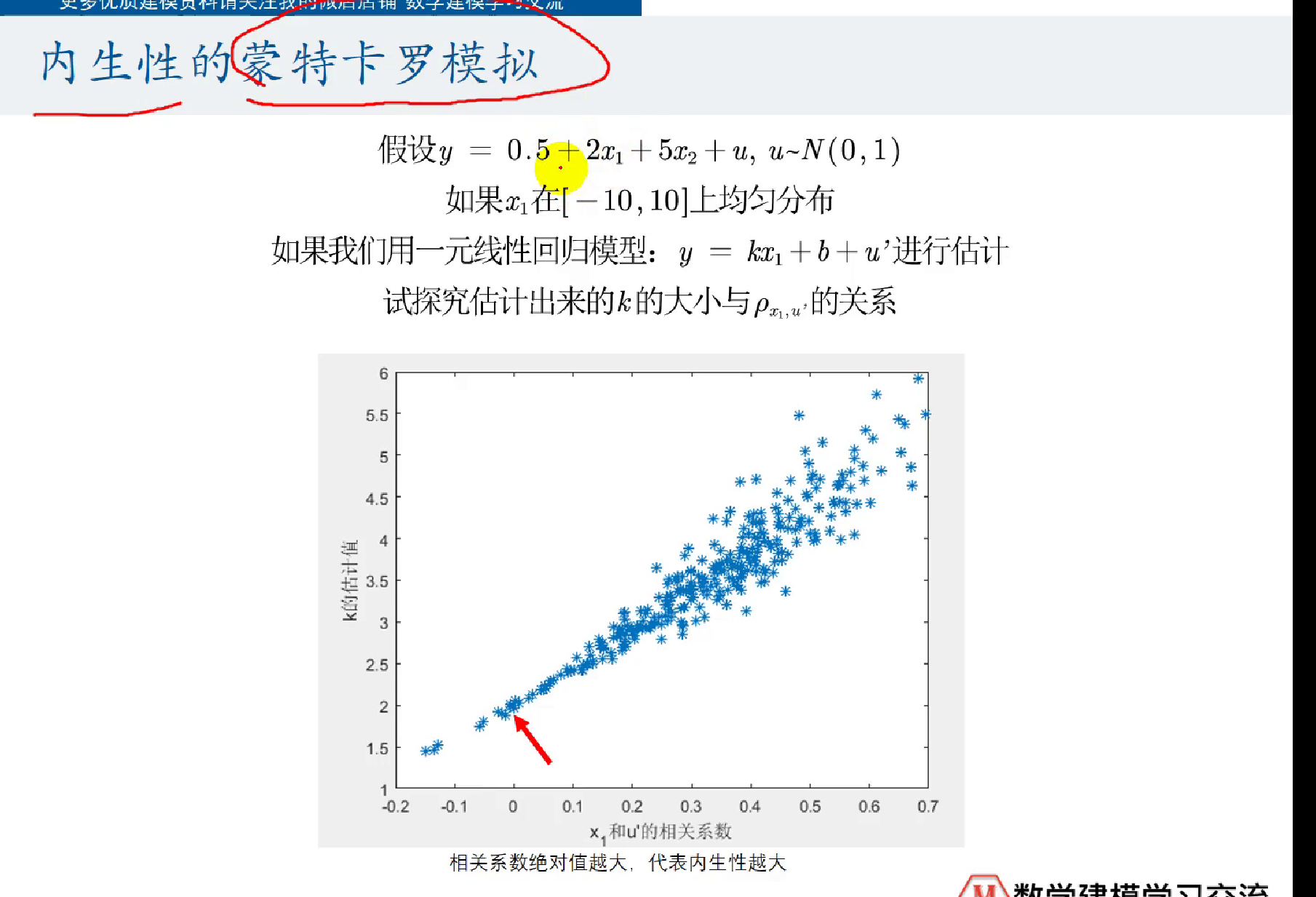matlab: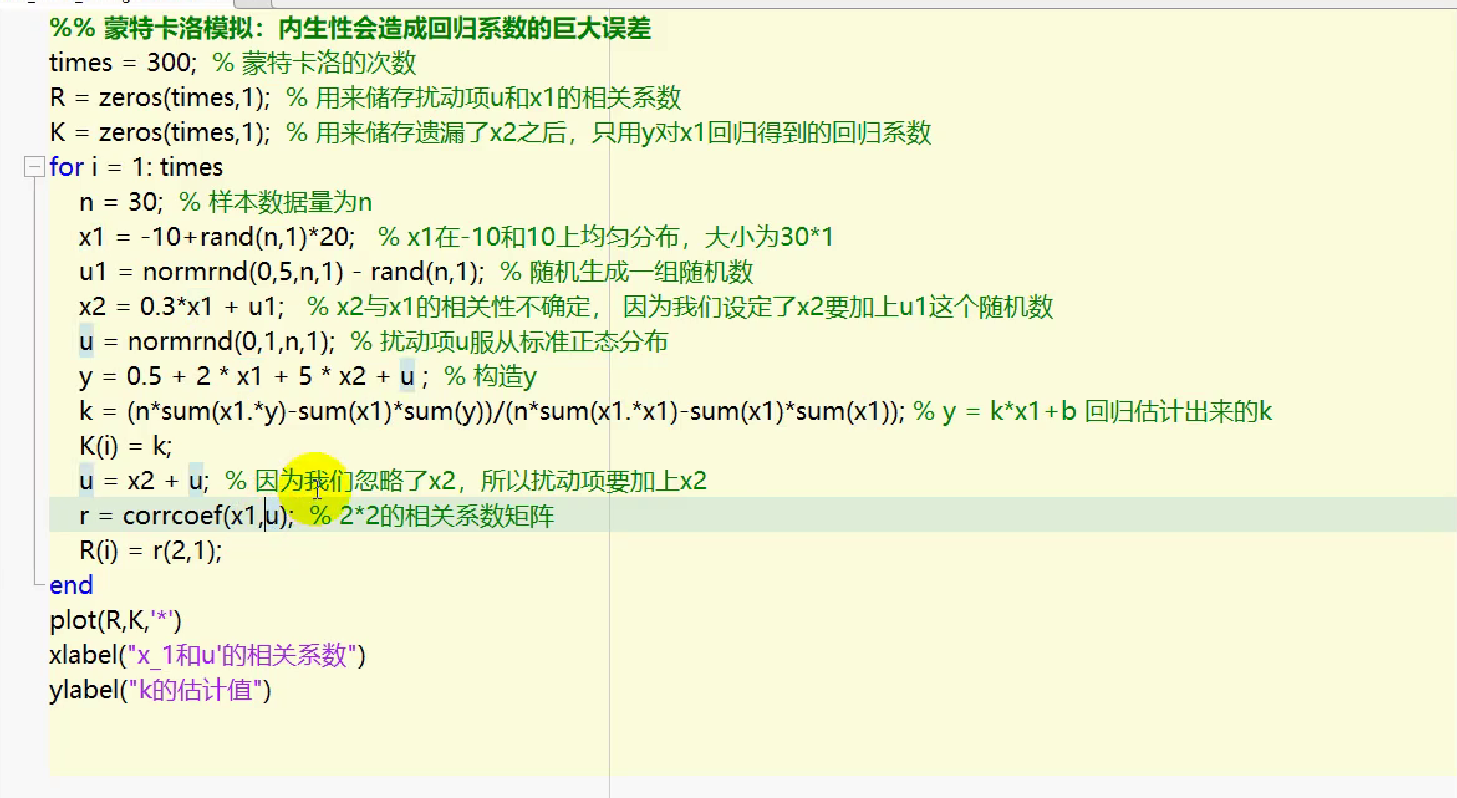降低内生性要求：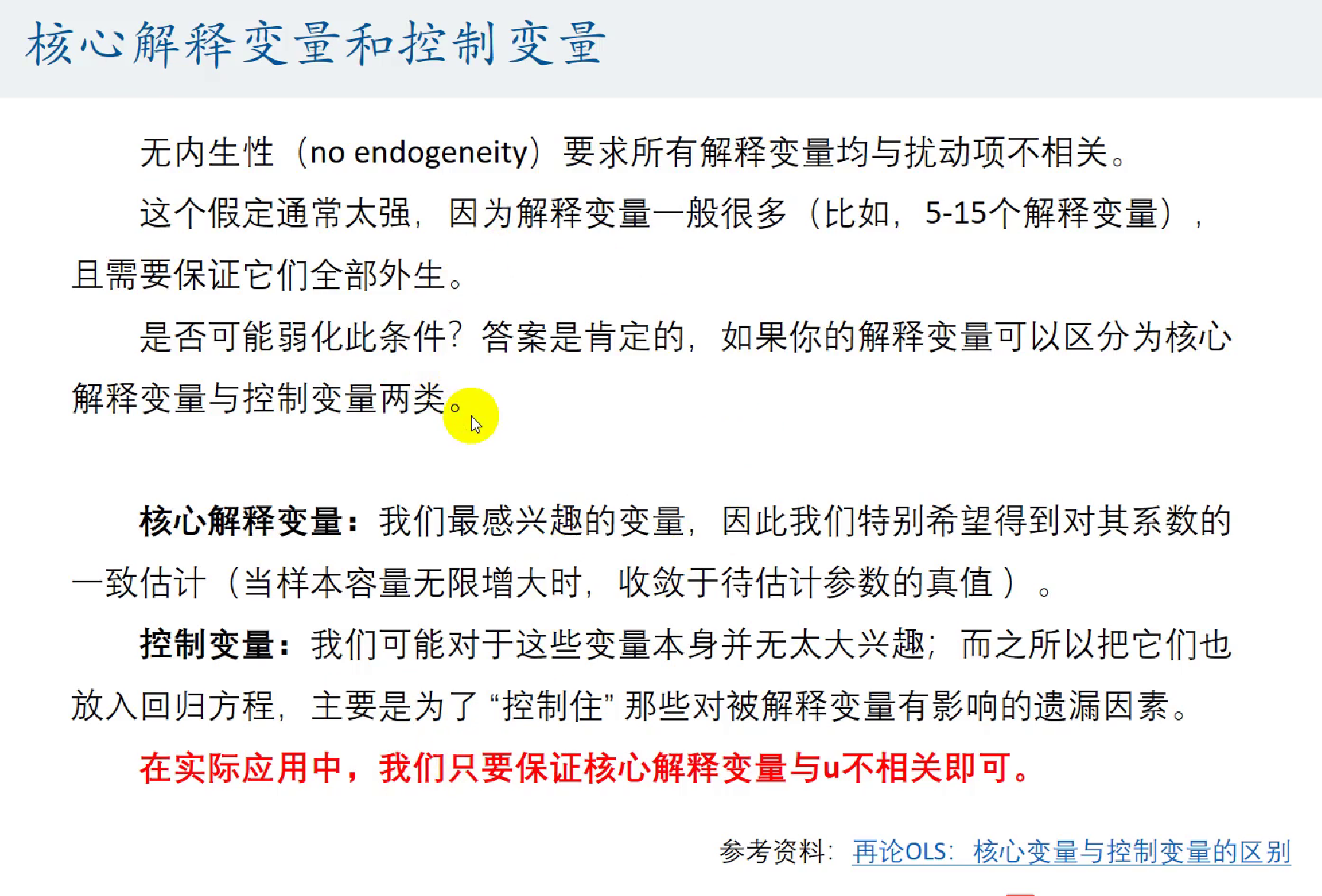展开全文数学建模
• 机器学习之多元线性回归模型梯度下降法的python实现 前言： 本文利用python实现多元线性回归模型的梯度下降算法，以二元线性回归模型为例，实现梯度下降算法，以及训练得到的三维图形结果展示。 一、二元线性回归...机器学习 梯度下降算法 python 二元线性回归
• 在上一篇文章中，我们分别研究了最小二乘估计量 β^OLS 和 σ^OLS 的相关性质，证明了 β^OLS 是 β 的一个最优线性无偏估计量（BLUE）， σ^2OLS 是 σ2 的一个无偏估计量，并得到了其在正态性误差假设下所对应的...
• 传统的多元线性回归模型可以用矩阵来描述。 按照OLS估计方法得出的多元线性回归的参数结果为 对于该式而言Y的估计值 其实正是n维向量Y 在n*k维矩阵（不存在向量自相关）所张成的k维空间上的正交投影。  ...
• （特殊的：自变量个数为1个，为一元线性回归）多元线性回归模型如下所示： 如上图所示，一元线性回归图形为一条直线。而二元线性回归，拟合的为一个平面。多元线性回归拟合出的图像为以超平面； 逻辑回归...机器学习
• 机器学习多元线性回归模型 如果有两个或两个以上的自变量，这样的线性回归分析就称为多元线性回归 实际问题中，一个现象往往是受多个因素影响的，所以多元线性回归比一元线性回归的实际应用更广 梯度下降法求解...算法 python 机器学习
• 衡量参数估计的指标2.1 无偏性2.2 一致性2.3 有效性3. 一些引理3.1 期望运算的线性性3.2 期望运算的线性性4. *β*^~OLS~ 的性质4.1 *β*^~OLS~ 服从的分布4.2 *β*^~OLS~ 与误差项之间的关系4.3 *β*^~OLS~ 的无偏...统计学 机器学习
• ## Python实现多元线性回归

万次阅读 多人点赞 2018-04-12 21:39:25
Python实现多元线性回归 线性回归介绍 线性回归是利用数理统计中回归分析，来确定两种或两种以上变量间相互依赖的定量关系的一种统计分析方法，运用十分广泛。其表达形式为y = w'x+e，e为误差服从均值为0的...Python 数据分析 数据挖掘 pandas
• 之前上学时计量经济学的模型实现总是用Eviews等...多元线性回归和显著性检验（参数估计、T检验、F检验、拟合优度） 多重共线性（导致结果、检验——方差膨胀因子、补救措施——岭回归） 异方差（导致结果、检验——W...统计学 数学建模 python
• 最近再做一些多元回归分析方面的分析，但对于得出的回归模型的好坏不知道如何才判断，于是查找了一下相关的教材书籍，找到了张文彤老师写的《SPSS统计分析高级教程》这本书，里面对于回归模型的优劣评价给出来了几点...
• 3.1多元线性回归模型 一、多元线性回归模型多元线性回归模型的一般形式为： Y=β0+β1*X1+β2*X1+⋯+βk*X1+μ 其中k为解释变量的数目, βj (j=0,1,⋯,k)称为回归系数。上式也被称为总体随机函数的随机表达形式...计量经济学 统计学
• TensorFlow实践（5）——多元线性回归模型（一）前 言（二）数据展示（三）模型的TensorFlow实现（1）模型参数设置（2）输入数据（3）构建模型（4）定义损失函数（5）选择优化器及定义训练操作（6）创建会话进行训练...TensorFlow 人工智能 机器学习
• 一、模型假设 传统多元线性回归模型 最重要的假设的原理为： 1. 自变量和因变量之间存在多元线性关系，因变量y能够被x1,x2….x{k}完全地线性解释；2.不能被解释的部分则为纯粹的无法观测到的误差 其它假设主要为： 1...
• 多元线性回归程序示例 类似的，我们也可以实现多元线性回归。这里，我们需要创建多个特征（x），我们也可以像之前程序那样，随机生成多个特征，不过，这里，我们使用sklearn库提供的更方面的方法。 from sklearn....可视化 机器学习
• 多重共线性指自变量问存在线性相关关系，即一个自变量可以用其他一个或几个自变量的线性表达式进行表示。若存在多重共线性，计算自变量的偏回归系数...那么当发现多重线性回归模型中存在多重共线性时我们该如何处理呢
• F检验 对于多元线性回归模型，在对每个回归系数进行显著性检验之前，应该对回归模型的整体做显著性检验。这就是F检验。当检验被解释变量yt与一组解释变量x1, x2 , ... , xk -1是否存在回归关系时，给出的零假设...
• 点击上方“Python爬虫与数据挖掘”，进行关注回复“书籍”即可获赠Python从入门到进阶共10本电子书今日鸡汤寥落古行宫，宫花寂寞红。前言「多元线性回归模型」非常常见，是大多数人入...机器学习 python 人工智能 数据分析 数据挖掘
• ## 多元线性回归

千次阅读 2019-12-29 21:45:18
二、多元线性回归模型 1.建立模型 以二元线性回归模型为例 ，二元线性回归模型如下： 类似的使用最小二乘法进行参数估计 ： 2.拟合优度指标 标准误差：对y值与模型估计值之间的离差的一种度量。其计算公式为： 3....
• 基于MATLAB的多元线性回归模型。文章充分利用 MATLAB统计工具箱的优势 ,通过程序的实现 ,对多元线性回归模型的未知参数估计方法以及对估 计后的模型预报做出研究 ,并以实例验证了该方法的有效性。
• SPSS--回归-多元线性回归模型案例解析！(一）  多元线性回归，主要是研究一个因变量与多个自变量之间的相关关系，跟一元回归原理差不多，区别在于影响因素（自变量）更多些而已，例如：一元线性回归方程 为： ...
• 多重线性回归 多元线性回归Video Link 影片连结 We have taken a look at Simple Linear Regression in Episode 4.1 where we had one variable x to predict y, but what if now we have multiple variables, not ...python 机器学习 人工智能 算法
• 多元线性回归:这是一种线性回归的形式，当有两个或多个预测因子时使用。 我们将看到多个输入变量如何共同影响输出变量，同时还将了解计算与简单LR模型的不同之处。我们还将使用Python构建一个回归模型。 最后，我们...算法 机器学习 人工智能机器学习 算法 最小二乘法
• 多元线性回归，主要是研究一个因变量与多个自变量之间的相关关系，跟一元回归原理差不多，区别在于影响因素（自变量）更多些而已，例如：一元线性回归方程 为： 毫无疑问，多元线性回归方程应该为： 上图中的 x1,......